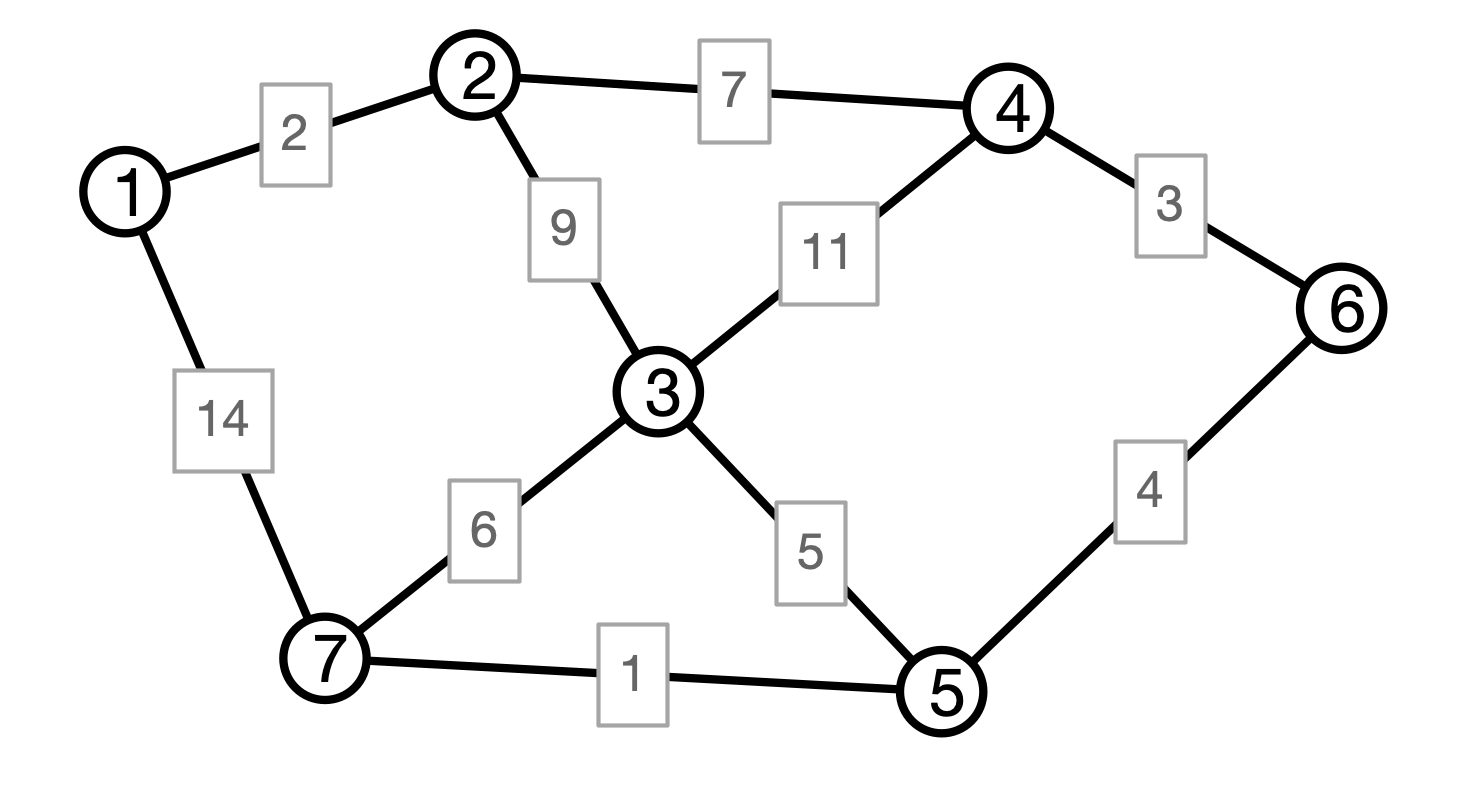# Lecture 19: Minimum Spanning Trees, Part 2

### COSC 311 Algorithms, Fall 2022

$\def\compare{ {\mathrm{compare}} } \def\swap{ {\mathrm{swap}} } \def\sort{ {\mathrm{sort}} } \def\insert{ {\mathrm{insert}} } \def\true{ {\mathrm{true}} } \def\false{ {\mathrm{false}} } \def\BubbleSort{ {\mathrm{BubbleSort}} } \def\SelectionSort{ {\mathrm{SelectionSort}} } \def\Merge{ {\mathrm{Merge}} } \def\MergeSort{ {\mathrm{MergeSort}} } \def\QuickSort{ {\mathrm{QuickSort}} } \def\Split{ {\mathrm{Split}} } \def\Multiply{ {\mathrm{Multiply}} } \def\Add{ {\mathrm{Add}} } \def\cur{ {\mathrm{cur}} } \def\gets{ {\leftarrow} }$

## Overview

2. Prim’s Algorithm, Again
3. Kruskal’s Algorithm

2. Very happy with grade distribution
3. Two more consistent issues
• Question 2 ($0$, $\Theta$, $\Omega$)
• Question 3 (D&C Algorithm)

## MSTs

Input:

• a weighted graph $G = (V, E)$ with edge weights $w$

Output:

• a set $F$ of edges in $E$ such that

1. $(V, F)$ is connected
2. sum of weights of edges in $F$ is minimal among all connected sub-graphs of $G$

The graph $T = (V, F)$ is called a minimum spanning tree of $G$

## Prim’s Algorithm

  PrimMST(V, E):
initialize set S = {v} with v arbitrary
initialize set F = {} of MST edges, priority queue Q
for each neighbor x of v
add (v, x) to Q with priority w(v, x)
while Q is not empty
(u, v) <- removeMin(Q)
if S doesn't contain v
for each neighbor x of v
add (v, x) to Q with priority w(v, x)
return (S, F)


## Prim Illustration## Cuts in Graphs

Definition. Let $G = (V, E)$ be a graph. A cut in $G$ is a partition of $V$ into two (non-empty) subsets $U$ and $V - U$.## Cuts and MSTs

Cut Claim. Suppose:

• $G = (V, E)$ is a weighted graph (with distinct edge weights)
• $U, V - U$ a cut in $G$
• $T = (V, F)$ an MST
• $e = (u, v)$ is the minimum weight edge that crosses the cut
• $u \in U$ and $v \in V - U$

Then:

• $T$ contains the edge $e$

## Cut Claim Illustration## Prim, Again

  PrimMST(V, E):
initialize set S = {v} with v arbitrary
initialize set F = {} of MST edges, priority queue Q
for each neighbor x of v
add (v, x) to Q with priority w(v, x)
while Q is not empty
(u, v) <- removeMin(Q)
if S doesn't contain v
for each neighbor x of v
add (v, x) to Q with priority w(v, x)
return (S, F)


## Prim Correctness I

Cut claim $\implies$ Prim produces an MST.

Why?

Consider $k$th edge $e_k$ added by Prim

• $S_k =$ contents of $S$ before edge added

What can we say about the cut $S_k, V - S_k$?

## Prim Correctness II

First Conclusion. Every edge $e$ added by Prim’s algorithm is in the MST.

Still to show. All MST edges are added.

Why is this so?

• Suffices to argue that Prim produces a spanning tree
• Set of edges found by Prim form a tree
• All vertices of $V$ are in tree (if $G$ is connected)

Conclusion. Prim’s algorithm produces an MST.

## Prim Running Time?

  PrimMST(V, E):
initialize set S = {v} with v arbitrary
initialize set F = {} of MST edges, priority queue Q
for each neighbor x of v
add (v, x) to Q with priority w(v, x)
while Q is not empty
(u, v) <- removeMin(Q)
if S doesn't contain v
for each neighbor x of v
add (v, x) to Q with priority w(v, x)
return (S, F)


## Conclusion

Prim’s algorithm:

• computes an MST in $G$
• if efficient priority queue is used, running time is $O(m \log n)$

Prim’s algorithm is greedy

• to grow a tree, always add the lightest outgoing edge

## MSTs, Another Way

Prim:

• Grow tree greedily from a single seed vertex
• Maintain a (connected) tree

Edge Centric View:

• Maintain a collection of edges (not necessarily a tree)
• Add edges to collection to eventually build an MST

Questions:

• How to prioritize edges?
• How to determine whether or not to include an edge?

## Picture## Kruskal’s Algorithm

  Kruskal(V, E, w):
C <- collection of components
initially, each vertex is own component
F <- empty collection
# iterate in order of increasing weight
for each edge e = (u, v) in E
if u and v are in different components then
merge components containing u and v
endif
endfor
return F


## Kruskal Illustration## Kruskal Correctness I

Claim 1. Every edge added by Kruskal must be in every MST.

Why?

• Suppose $e = (u, v)$ added by Kruskal
• Consider the cut $U, V - U$ where $U$ is $u$’s component
• $e$ is lightest edge across the cut (why?)
• therefore $e$ must be in MST (why?)

## Kruskal Correctness II

Claim 2. Kruskal produces a spanning tree.

Why?

• edges added by Kruskal do not contain cycles (why?)

• edges edges added by Kruskal connect graph (why?)

## Conclusion

Theorem. Kruskal’s algorithm produces an MST.

Question. How could we implement Kruskal’s algorithm efficiently? What is its running time?

## Next Time

1. Efficiency of Kruskal
2. Interval Scheduling